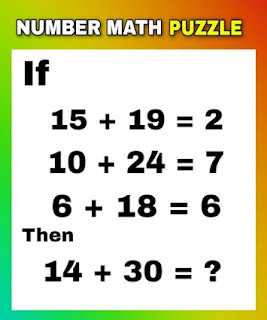# GkJobs

GkJobs

IF
15 + 19 = 2
10 + 24 = 7
6 + 18 = 6
THEN
14 + 30 = ?So were you able to guess the answer? if yes then congratulation if no don't worry scroll down further to know the right answer.

EXPLANATION-:

EQUATION 1 -: ( 19 - 15 ) / 2 = 2

EQUATION 2 -: ( 24 - 10 ) / 2 = 7

EQUATION 3 -: ( 18 - 6 ) / 2 = 6

EQUATION 4 -: ( 30 - 14 ) / 2 = 8

Thanks for visiting our website
Have a good day
Tag : Online Test